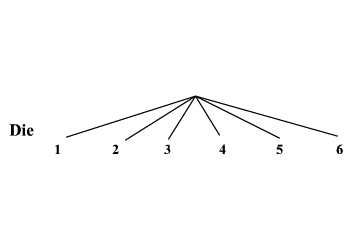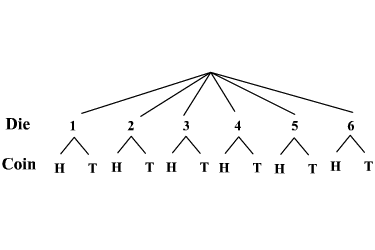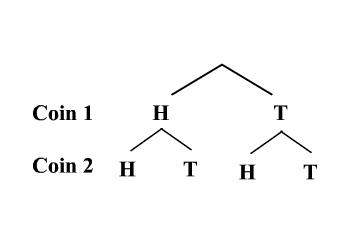# Compound Events

In probability , while a simple event consists of exactly one outcome, a compound event is a combination of two or more simple events. For example, tossing a coin is a simple event, but rolling a die and tossing a coin is a combination of $2$ simple events and therefore a compound event.

The probability of a simple event is defined as the ratio of the number of favorable outcomes to the total number of possible outcomes. For example, consider the probability of getting an even number when a six-sided die is rolled. There are six possible outcomes— $1,2,3,4,5$ and $6$ —and three favorable ones: $2,4$ and $6$ are even numbers. So, the probability is $\frac{3}{6}$ , or $\frac{1}{2}$ after we reduce the fraction.

The probability of a compound event is defined in the same way. However, finding all the possible outcomes in the sample space can be a bit more complicated.

The sample space for a compound event can be represented in three different ways: organized lists, tree diagrams, and tables.

In an organized list, you simply list all the possible outcomes that could occur. For example, if you roll a die and flip a coin, what is the probability of getting a number less than $4$ and heads?

Consider all the possible outcomes of the compound event. The possible outcomes of rolling a die are $1,2,3,4,5$ and $6$ , and the possible outcomes of flipping a coin are heads and tails. So, if we use the notation $1-\text{H}$ to indicate a $1$ on the die and heads on the coin, the sample space of the compound event consists of the following outcomes.

$1-\text{H},2-\text{H},3-\text{H},4-\text{H},5-\text{H},6-\text{H},1-\text{T},2-\text{T},3-\text{T},4-\text{T},5-\text{T}$ and $6-\text{T}$

Among these $12$ outcomes, the favorable ones are $1-\text{H}$ , $2-\text{H}$ and $3-\text{H}$ . That is, the probability of getting a number less than $4$ and heads is $\frac{3}{12}$ or $\frac{1}{4}$ .

Example 1:

Raphael has a quarter, a dime, and a nickel in his left pocket. In his right pocket he has a blue marble and a red marble. If he selects a coin from his left pocket and a marble from his right, what is the probability that he is holding EITHER a dime OR a blue marble?

List all the possible outcomes of the compound event. Use $\text{Q-B}$ to mean "Quarter and Blue".

$\text{Q-B},\text{Q-R},\text{D-B},\text{D-R},\text{N-B},\text{N-R}$

Out of these $6$ outcomes, the favorable ones are $\text{Q-B},\text{D-B},\text{N-B}$ and $\text{D-R}$ . That is, the probability of getting a dime or a blue marble is $\frac{4}{6}$ or $\frac{2}{3}$ .

Another way to represent the sample space of a compound event is to use tree diagrams. Consider the example of rolling a die and flipping a coin. The outcomes of the first event—say, rolling a die—can be represented as the first set of branches of the tree diagram.Now, the possible outcomes of the second event can be drawn as the sub-branch of each of the branches of the first event.Note that the order in which the events are considered is immaterial. That is, whether you consider the event of flipping the coin as first or second will not make any difference to the sample space.

Example 2:

What is the probability of tossing two coins simultaneously and getting exactly one tail? The possible outcomes for each of the coins are head and tail. Starting with the outcomes of one of the coins, we can draw the tree diagram as shown.Counting all the branch-endings of the tree diagram, the total number of possible outcomes is $4$ . Among these the favorable outcomes are the ones with one tail each. That is, the number of favorable outcomes is $2$ . Therefore, the probability of the compound event is $\frac{2}{4}$ or $\frac{1}{2}$ .

A third way to represent a sample space is using a table.

Let us again consider the compound event of rolling a die and flipping a coin. The outcomes of the event of rolling a die can be listed on top and the event of flipping a coin can be can be listed on the side as shown.

 Head Tail $\text{1}$ $\text{1-H}$ $\text{1-T}$ $\text{2}$ $\text{2-H}$ $\text{2-T}$ $\text{3}$ $\text{3-H}$ $\text{3-T}$ $\text{4}$ $\text{4-H}$ $\text{4-T}$ $\text{5}$ $\text{5-H}$ $\text{5-T}$ $\text{6}$ $\text{6-H}$ $\text{6-T}$

The probability of the event calculated remains the same.

Example 3:

In her bookshelf, Johanna has five math books, numbered from $1$ to $5$ , on the first shelf, and six puzzles, labeled $\text{A}$ to $\text{F}$ , on the second shelf. If she chooses a book and a puzzle at random, what is the probability of getting a book with an odd number and puzzle labeled $\text{D}$ ?

The outcomes of the event of picking a book can be listed on top and the event of picking a puzzle can be listed on the side as shown.

 $\text{1}$ $\text{2}$ $\text{3}$ $\text{4}$ $\text{5}$ $\text{A}$ $\text{A-1}$ $\text{A-2}$ $\text{A-3}$ $\text{A-4}$ $\text{A-5}$ $\text{B}$ $\text{B-1}$ $\text{B-2}$ $\text{B-3}$ $\text{B-4}$ $\text{B-5}$ $\text{C}$ $\text{C-1}$ $\text{C-2}$ $\text{C-3}$ $\text{C-4}$ $\text{C-5}$ $\text{D}$ $\text{D-1}$ $\text{D-2}$ $\text{D-3}$ $\text{D-4}$ $\text{D-5}$ $\text{E}$ $\text{E-1}$ $\text{E-2}$ $\text{E-3}$ $\text{E-4}$ $\text{E-5}$ $\text{F}$ $\text{F-1}$ $\text{F-2}$ $\text{F-3}$ $\text{F-4}$ $\text{F-5}$

Among these $30$ outcomes, the favorable ones are $\text{D-1},\text{D-3}$ and $\text{D-5}$ . Therefore, the probability is $\frac{3}{30}$ or $\frac{1}{10}$ .